# Pandas DataFrame by Example

Last updated:

Pandas is a very versatile tool for data analysis in Python and you must definitely know how to do, at the bare minimum, simple operations on it.

View this notebook for live examples of techniques seen here

Here are a couple of examples to help you quickly get productive using Pandas' main data structure: the DataFrame

Updated for version: 0.20.1

So here are some of the most common things you'll want to do with a DataFrame:

## Read CSV file into DataFrame

# needed all around
import pandas as pd

# the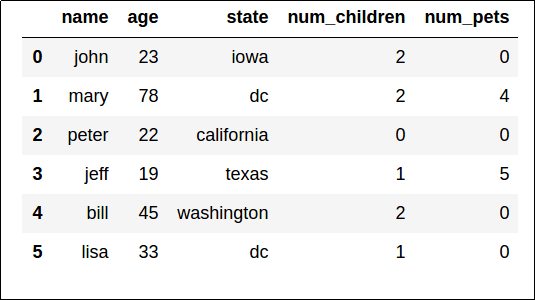This is what our sample dataset looks like

To use a column in the file as the dataframe index, use index_col argument:

import pandas as pd

# note that Pandas will NOT warn you if the column you've selected
# is NOT unique!


## Write DataFrame into CSV file

# simplest possible usage
df.to_csv("data-out.csv")


Omit the index column for a cleaner CSV file:

df.to_csv("data-out-no-index.csv", index=False)


## Select rows by position

Just use iloc. Position starts at 0.

The same rules apply as in regular python list slicing

# select the first 2 rows
df.iloc[:2]

# select the last 2 rows
df.iloc[-2:]


## Select Rows by index value

Just use loc.

compare this with iloc above

# select rows up to and including the one
# with index=2 (this retrieves 3 rows)
df.loc[:2]


## Select rows by column value

# people whose "age" is greater than 30
df[df["age"] > 30]

# people who have more pets than children
df[ df["num_pets"] > df[ "num_children"] ]


## Select rows by multiple column values

# people older than 40 who own pets
df[ (df["age"] > 40) & (df["num_pets"] > 0) ]


## Select columns starting with

Select all columns starting with 'n':

df[[colname for colname in df.columns if colname.startswith('n')]]


## Select all columns but one

Use df.drop(). Note the axis=1 parameter.

Also, the columns must be passed as a list (even if it's a single column you want to exclude from the selection).

# df itself is not modified; a copy is returned instead
df.drop(["age","num_children"],axis=1)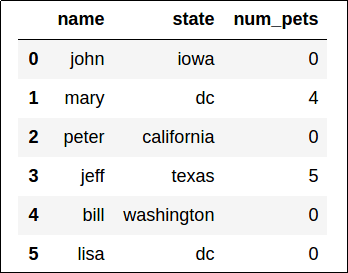A new dataframe is returned, with columns "age" and "num_children" removed.

## Apply an aggregate function to every column

For every numeric column, what is the average over all rows?

Note that our resultset contains 3 rows (one for each numeric column in the original dataset).

df[["age","num_pets","num_children"]].apply(lambda row: np.mean(row),axis=0)
# >>
# age             36.666667
# num_pets         1.500000
# num_children     1.333333


## Apply an aggregate function to every row

The same as above, but note that axis=1 is used.

For every row, what is the sum of all numeric columns?

# note that our resultset will have 6 rows (one for every row in the
# original dataset)
df[["age","num_pets","num_children"]].apply(lambda row: np.sum(row),axis=1)
# >>
# 0    25
# 1    84
# 2    22
# 3    25
# 4    47
# 5    34


## Transform dataframe

Remember: df[['colname']] returns a new DataFrame, while df['colname'] returns a Series

For numerical data, it's straighforward:

this is requivalent to df[["age"]] * 2

# returns a new dataframe where every age
# is double its old value
df[["age"]].apply(lambda value: value*2)


For text data (or otherwise non-numerical) you need to cast:

# returns a new dataframe where every name is
# the old name to uppercase
df[["name"]].apply(lambda value: value.str.upper())


## Shuffle rows in DataFrame

Method reindex() can be used to reindex your data and, if you pass random indices, you'll have shuffled your data:

# using a random permutation of the original indices
df = df.reindex(np.random.permutation(df.index))


## Iterate over all rows in a DataFrame

Using for ... in. This is similar to iterating over Python dictionaries (think iteritems() or items()in Python 3):

for index,row in df.iterrows():
print("{0} has name: {1}".format(index,row["name"]))
# >>
# 0 has name: john
# 1 has name: mary
# 2 has name: peter
# 3 has name: jeff
# 4 has name: bill
# 5 has name: lisa


## Randomly sample rows from DataFrame

Sampling is commonly used in Machine Learning tasks and many others.

# sample 4 rows from df
random_indices = np.random.choice(df.index.values, 4, replace=False)

# iloc retrieves rows by position, but the dataframe is now smaller
# so use loc instead (loc retrieves rows by their numeric indices)
sampled_df = df.loc[random_indices]


## Sort DataFrame by column value

This is pretty self-explanatory:

# sort by "age" column, larger to smaller
df.sort_values("age",ascending=False)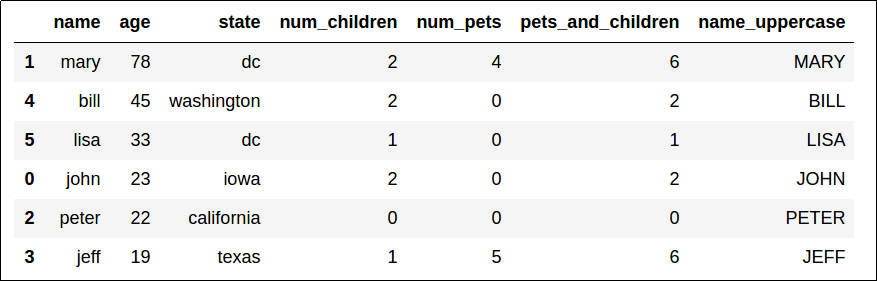Sorted by "age", descending.

You can also use multiple columns to break ties:

# sort by column "num_pets" descending and in case there
# are ties, use "age" ascending to sort those
df.sort_values( ["num_pets","age"], ascending=[False,True] )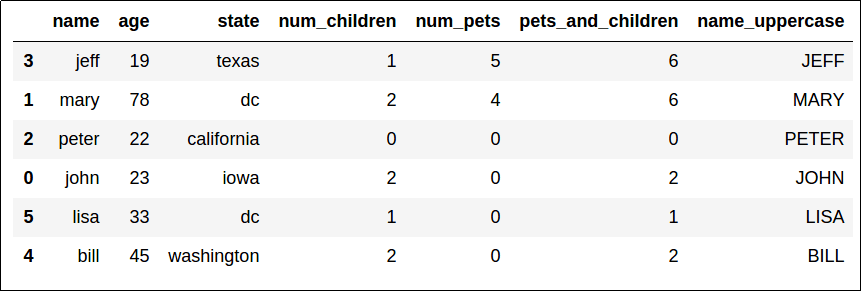Sorted by "num_pets" (descending), then by "age" (ascending) to break ties.

## Custom sort

Your boss has asked you to sort records by age, but put everybody with "N/A" for state at the end.

One way to solve this is to create a new column rank and use that in sorting:

import pandas as pd

df = pd.DataFrame({
'name':['john','mary','peter','jeff','bill','lisa'],
'age':[23,78,22,19,12,33],
'state':['N/A','dc','california','texas','N/A','dc']
})

# use this function to convert a state string to 0 or 1
def state_to_rank(state):
if state=="N/A":
return 1
else:
return 0

df['rank'] = df['state'].map(lambda x: state_to_rank(x))

df.sort_values(by=['rank','age'])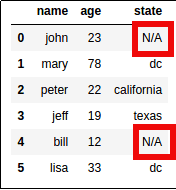The real world has
lots of missing data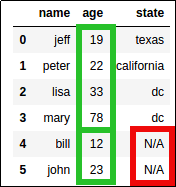Ages are sorted in each group

## Select rows using lambdas

If you need something more complex that the regular df[df['somecolumn'] == 'somevalue'], you can use apply with a lambda function too:

# select rows whose name begins with the letter 'j'
df[df.apply(lambda row: row['name'].startswith('j'),axis=1)]Filter only rows where column "name" starts with 'j'

## Split a dataframe by column value

grouped = df.groupby(df["num_pets"])

grouped.groups.keys()
# [0,4,5]

# this is a dataframe containing only data for people
# who have zero pets
df0 = grouped.get_group('0')


## Apply multiple aggregation operations on a single GroupBy pass

Say, for instance, ORDER_DATE is a timestamp column. We want to find out the total quantity QTY AND the average UNIT price per day.

Note: we're not using the sample dataframe here

grouped = df.groupby(p7.ORDER_DATE.dt.day).agg({
"QTY":np.sum,
"UNIT": np.mean
})


## Verify that the dataframe includes specific values

This is done using the .isin() method, which returns a boolean dataframe to indicate where the passed values match.

# if the method is passed a simple list, it matches
# those values anywhere in the dataframe
df.isin([2,4])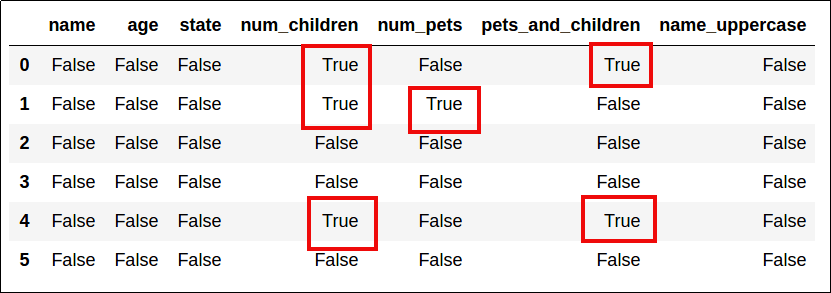Elements that match the values in the original dataframe become True

# you can also pass a dict or another dataframe
# as argument
df.isin({'num_pets':[4,5]})When a dict is passed, columns must match the dict keys too.